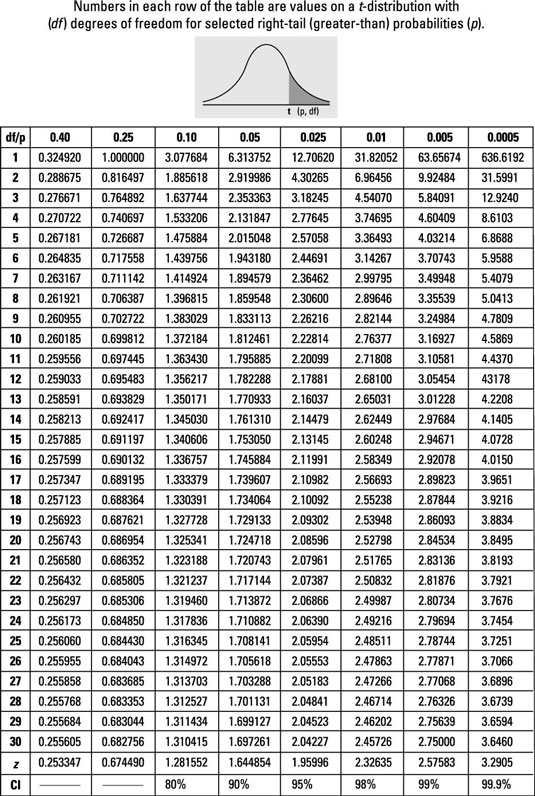## 24.3 The t-table• The t-table (for the t-distribution) is different from the Z-table (for the Z-distribution)

• In the t-table (on the right) numbers in each row are values on a $$t$$-distribution with df for selected right-tail probabilities.

• Finding probabilities for various t-distributions, using the t-table, is a valuable statistics skill.